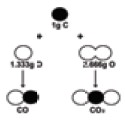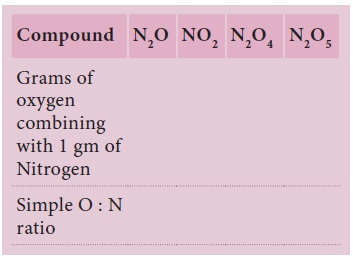Home | | Science 9th std | Laws of Chemical combination

# Laws of Chemical combination

Science : Atomic Structure : Laws of Chemical combination

Laws of Chemical combination

Out of these five laws you already know the first two laws. Let us see the next three laws in detail in this chapter.

## 1. Law of multiple proportions

This law was proposed by John Dalton in 1804.To illustrate the law let us consider the following example.

Carbon combines with oxygen to form two different oxides, carbon monoxide(CO) and carbon dioxide (CO2).The ratio of masses of oxygen in CO and CO2 for xed mass of carbon is 1: 2. Isnâ€™t this a simple ratio? Let us take one more example. Sulphur combines with oxygen to form sulphur dioxide and sulphur trioxide. The ratio of masses of oxygen in SO2 and SO3 for xed mass of Sulphur is 2:3.### Sample Problem (Solved)

Iron forms two different chlorides, namely ferrous and ferric chlorides. Each of these chlorides was prepared from 2 gram of iron. It was found that 4.538 gram ferrous chloride and 5.804 gram ferric chloride were produced. Show that these observations are according to the law of multiple proportions.

Solution:

Here iron is forms different chlorides. The weight of iron taken in both cases is the same. i.e. 2.0 g. Therefore, we haveThe proportion of chlorine in this compound isThe proportion by weight of chlorine is indicated by a simple ratio. Thus Law of multiple proportions is verifiedTags : Chemistry , 9th Science : Atomic Structure
Study Material, Lecturing Notes, Assignment, Reference, Wiki description explanation, brief detail
9th Science : Atomic Structure : Laws of Chemical combination | Chemistry